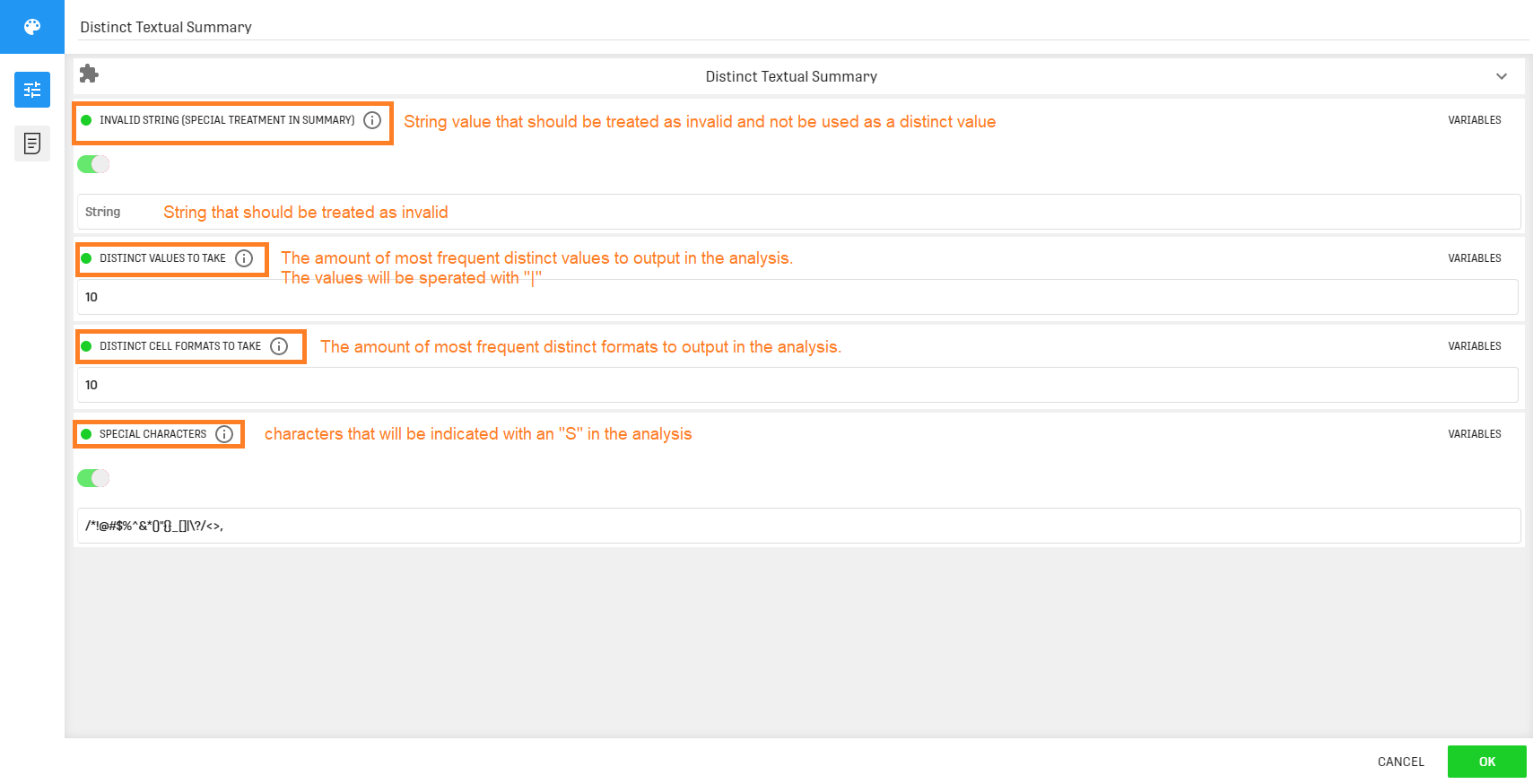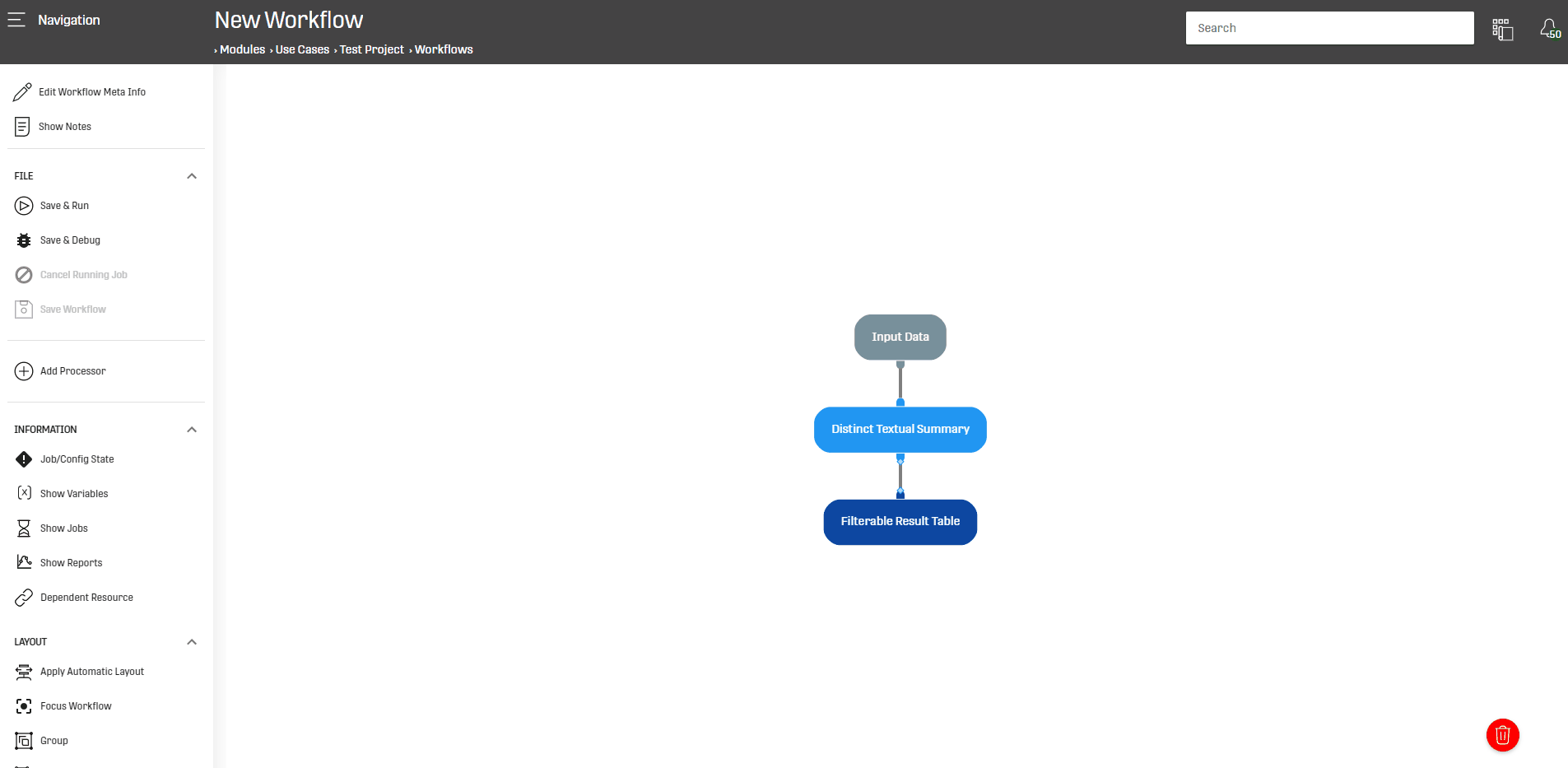# Overview

This processor creates textual descriptive summaries for every column in the dataset. Statistics include most frequent values, most frequent patterns (value formats, e.g. number, uppercase and lowercase combinations), amount of invalid rows (invalid value can be specified) and valid rows, amount of distinct values as well as minimum, mean and maximum value length (for textual representations).

# Configuration

This processor takes any Dataset as input. The configuration interface looks as follows:# Output

This processor computes statistical summaries for each column of the input Dataset, and gives the following output columns:

• Column_Name: the name of the column in the input Dataset.
• Nr_Distinct_Values: number of distinct values in each column.
• Most_Frequent_Distinct_Values: Uses the value in the second configuration field (Distinct Values to take) to output the most frequent values along with their frequencies (values are separated with "|")
• Invalid: number of invalid entries in a column.
• Total: total number of values within a column.
• Nr_Characters: average number of characters for each value in a column.
• Min_Nr_Characters: minimum number of characters in a value.
• Max_Nr_Characters: maximum number of characters in a value.
• Column_Formats: displays the formats existent in the different columns ("l": for alphabet in lower case, "L" upper case,, "o": operator,  "n": for number, "S" for special characters that are defined within the last configuration field).
• Most_Frequent_Column_Format: The most occurring format.

# Example

In this example the Distinct Textual Summary Processor will be applied on a simple Dataset to extract some textual statistics from it:# Related Articles

Distinct Summary Processor

Heuristic Summary Processor